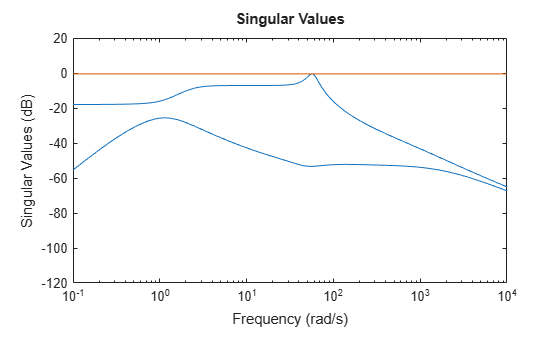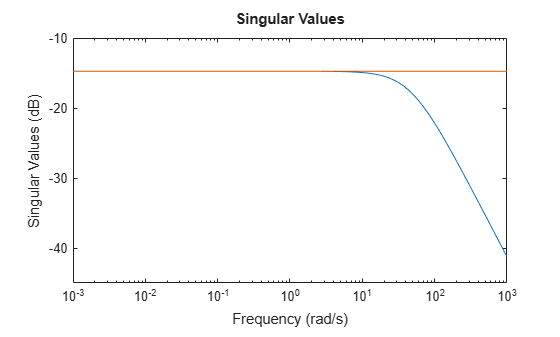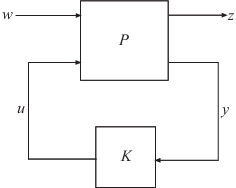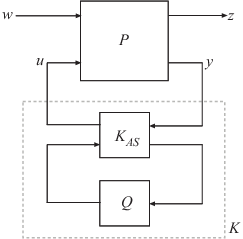# hinfsyn

Compute H-infinity optimal controller

## Syntax

``[K,CL,gamma] = hinfsyn(P,nmeas,ncont)``
``[K,CL,gamma] = hinfsyn(P,nmeas,ncont,gamTry)``
``[K,CL,gamma] = hinfsyn(P,nmeas,ncont,gamRange)``
``[K,CL,gamma] = hinfsyn(___,opts)``
``[K,CL,gamma,info] = hinfsyn(___)``

## Description

example

````[K,CL,gamma] = hinfsyn(P,nmeas,ncont)` computes a stabilizing H∞-optimal controller `K` for the plant `P`. The plant has a partitioned form$\left[\begin{array}{c}z\\ y\end{array}\right]=\left[\begin{array}{cc}{P}_{11}& {P}_{12}\\ {P}_{21}& {P}_{22}\end{array}\right]\left[\begin{array}{c}w\\ u\end{array}\right],$where: w represents the disturbance inputs.u represents the control inputs.z represents the error outputs to be kept small.y represents the measurement outputs provided to the controller.`nmeas` and `ncont` are the number of signals in y and u, respectively. y and u are the last outputs and inputs of `P`, respectively. `hinfsyn` returns a controller `K` that stabilizes `P` and has the same number of states. The closed-loop system `CL`` = lft(P,K)` achieves the performance level `gamma`, which is the H∞ norm of `CL` (see `hinfnorm`).```

example

````[K,CL,gamma] = hinfsyn(P,nmeas,ncont,gamTry)` calculates a controller for the target performance level `gamTry`. Specifying `gamTry` can be useful when the optimal controller performance is better than you need for your application. In that case, a less-than-optimal controller can have smaller gains and be more numerically well-conditioned. If `gamTry` is not achievable, `hinfsyn` returns `[]` for `K` and `CL`, and `Inf` for `gamma`. ```

example

````[K,CL,gamma] = hinfsyn(P,nmeas,ncont,gamRange)` searches the range `gamRange` for the best achievable performance. Specify the range with a vector of the form `[gmin,gmax]`. Limiting the search range can speed up computation by reducing the number of iterations performed by `hinfsyn` to test different performance levels. ```

example

````[K,CL,gamma] = hinfsyn(___,opts)` specifies additional computation options. To create `opts`, use `hinfsynOptions`. Specify `opts` after all other input arguments.```

example

````[K,CL,gamma,info] = hinfsyn(___)` returns a structure containing additional information about the H∞ synthesis computation. You can use this argument with any of the previous syntaxes.```

## Examples

collapse all

Synthesize a controller using different target performance levels. The plant in this example is based on the augmented plant model used in Robust Control of Active Suspension. Load the plant.

```load hinfsynExData P size(P)```
```State-space model with 5 outputs, 4 inputs, and 9 states. ```

This plant has five outputs and four inputs, where the last two outputs are measurement signals to provide to the controller, and the last input is a control signal. Compute an ${H}_{\infty }$-optimal controller.

```ncont = 1; nmeas = 2; [K1,CL,gamma] = hinfsyn(P,nmeas,ncont);```

The resulting two-input, one-output controller has the same number of states as P.

`size(K1)`
```State-space model with 1 outputs, 2 inputs, and 9 states. ```

The optimal performance level achieved by this controller is returned as `gamma`. This value is the ${H}_{\infty }$ norm of the closed-loop system `CL`.

`gamma`
```gamma = 0.9405 ```

You can examine the singular value plot of the closed-loop system to confirm that its largest singular value does not exceed `gamma`.

```sigma(CL,ss(gamma)) ylim([-120,20]);```For controllers that are close to optimal performance, controller gains can sometimes get large. If you know that your application does not require the optimal achievable performance level, you can limit the range of $\gamma$ values that `hinfsyn` tests. Suppose you know that $\gamma \approx 1.5$ is good enough for your application. Using the same plant as in the example H-Infinity Controller Synthesis, compute a controller using a target performance range of [1.4,1.6]. Turn on the display to see the progress of the computation.

```load hinfsynExData P ncont = 1; nmeas = 2; opts = hinfsynOptions('Display','on'); gamRange = [1.4 1.6]; [K,CL,gamma,info] = hinfsyn(P,nmeas,ncont,gamRange,opts);```
``` Test bounds: 1.4 <= gamma <= 1.6 gamma X>=0 Y>=0 rho(XY)<1 p/f 1.60e+00 4.9e-07 1.5e-21 1.462e-02 p 1.50e+00 5.0e-07 0.0e+00 1.681e-02 p 1.45e+00 5.0e-07 0.0e+00 1.803e-02 p 1.42e+00 5.0e-07 0.0e+00 1.868e-02 p 1.41e+00 5.0e-07 0.0e+00 1.902e-02 p Best performance (actual): 0.946 ```

The display shows all the performance levels tested by `hinfsyn`. In this case, all tested performance levels pass the tests that `hinfsyn` applies for closed-loop stability (see Algorithms). Although the smallest tested level is 1.41, the controller returned for that value achieves an actual performance level of `gamma`, which is about 0.95. The smallest tested level is returned in the `gamma` field of the `info` structure.

`info.gamma`
```ans = 1.4117 ```

If you try to obtain a performance level that is not achievable with any controller, the display informs you that the target is too small, and returns an empty controller and closed-loop system. For example, suppose you try to achieve a performance level of 0.75.

`gamTry = 0.75`
```gamTry = 0.7500 ```
`[K,CL,gamma] = hinfsyn(P,nmeas,ncont,gamTry,opts)`
```Specified upper limit GMAX=0.75 is too small, needs to be greater than 0.94. K = [] CL = [] ```
```gamma = Inf ```

Design a mixed-sensitivity controller for the following plant, augmented by the following loop-shaping filters (see `mixsyn`).

`$G\left(s\right)=\frac{s-1}{s+1},{W}_{1}=\frac{0.1\left(s+100\right)}{100s+1},{W}_{2}=0.1,no\phantom{\rule{0.2777777777777778em}{0ex}}{W}_{3}.$`

Define the plant, weighting filters, and augmented plant.

```s = zpk('s'); G = (s-1)/(s+1); W1 = 0.1*(s+100)/(100*s+1); W2 = 0.1; W3 = []; P = augw(G,W1,W2,W3);```

Synthesize the controller.

```[K,CL,gamma] = hinfsyn(P,1,1); gamma```
```gamma = 0.1831 ```

For this system, `gamma` is about 0.18, or about –15 dB.

Examine the singular values of the closed-loop result.

`sigma(CL,ss(gamma))`Compute a new controller for the same system with no ${W}_{1}$.

```W1 = []; P = augw(G,W1,W2,W3); [K,CL,gamma] = hinfsyn(P,1,1);```

In this case, the resulting controller `K` is zero, and the closed-loop transfer function `CL = K*(1+G*K)` is also zero.

## Input Arguments

collapse all

Plant, specified as a dynamic system model such as a state-space (`ss`) model. `P` can be any LTI model with inputs [w;u] and outputs [z;y], where:

• w represents the disturbance inputs.

• u represents the control inputs.

• z represents the error outputs to be kept small.

• y represents the measurement outputs provided to the controller.

Construct `P` such that measurement outputs y are the last outputs, and the control inputs u are the last inputs.

The function converts `P` to a state-space model of the form:

`$\begin{array}{c}dx=Ax+{B}_{1}w+{B}_{2}u\\ z={C}_{1}x+{D}_{11}w+{D}_{12}u\\ y={C}_{2}x+{D}_{21}w+{D}_{22}u.\end{array}$`

If `P` is a generalized state-space model with uncertain or tunable control design blocks, then `hinfsyn` uses the nominal or current value of those elements.

One application of H control is direct shaping of closed-loop singular value plots of control systems. In such applications, you augment the plant inputs and outputs with weighting functions (loop-shaping filters) that represent control objectives that you want the H controller to satisfy. For a detailed example that constructs such a partitioned, augmented plant for H synthesis, see Robust Control of Active Suspension. For further information, see Mixed-Sensitivity Loop Shaping.

#### Conditions on P

For the H synthesis problem to be solvable, (A,B2) must be stabilizable, and (A,C2) must be detectable. For the default Riccati method, the plant is further restricted in that P12 and P21 must have no zeros on the imaginary axis (continuous-time plants) or the unit circle (discrete-time plants). In continuous time, this restriction means that

`$\left[\begin{array}{cc}A-j\omega & {B}_{2}\\ {C}_{1}& {D}_{12}\end{array}\right]$`

has full column rank for all frequencies ω. By default, `hinfsyn` automatically adds extra disturbances and errors to the plant to ensure that the restriction on P12 and P21 is met. This process is called regularization. If you are certain your plant meets the conditions, you can turn off regularization using the `Regularize` option of `hinfsynOptions`.

Number of measurement output signals in the plant, specified as a nonnegative integer. The function takes the last `nmeas` plant outputs as the measurements y. The returned controller `K` has `nmeas` inputs.

Number of control input signals in the plant, specified as a nonnegative integer. The function takes the last `ncont` plant inputs as the controls u. The returned controller `K` has `ncont` outputs.

Target performance level, specified as a positive scalar. `hinfsyn` attempts to compute a controller such that the H of the closed-loop system does not exceed `gamTry`. If this performance level is achievable, then the returned controller has `gamma``gamTry`. If `gamTry` is not achievable, `hinfsyn` returns an empty controller.

Performance range for search, specified as a vector of the form `[gmin,gmax]`. The `hinfsyn` command tests only performance levels within that range. It returns a controller with performance:

• `gamma``gmin`, when `gmin` is achievable.

• `gmin` < `gamma` < `gmax`, when `gmax` is achievable and but `gmin` is not.

• `gamma` = `Inf` when `gmax` is not achievable. In this case, `hinfsyn` returns `[]` for `K` and `CL`.

If you know a range of feasible performance levels, specifying this range can speed up computation by reducing the number of iterations performed by `hinfsyn` to test different performance levels.

Additional options for the computation, specified as an options object you create using `hinfsynOptions`. Available options include:

• Display algorithm progress at the command line.

• Turn off automatic scaling and regularization.

• Specify an optimization method.

For information about all options, see `hinfsynOptions`.

## Output Arguments

collapse all

Controller, returned as a state-space (`ss`) model object or `[]`. The controller stabilizes `P` and has the same number of states as `P`. The controller has `nmeas` inputs and `ncont` outputs.

If you supply `gamTry` or `gamRange` and the specified performance values are not achievable, then `K` = `[]`.

Closed-loop transfer function, returned as a state-space (`ss`) model object or `[]`. The closed-loop transfer function is given by `CL = lft(P,K)` as in the following diagram.The returned performance level `gamma` is the H norm of `CL`.

If you supply `gamTry` or `gamRange` and the specified performance levels are not achievable, then `CL` = `[]`.

Controller performance, returned as a nonnegative scalar value or `Inf`. This value is the performance achieved using the returned controller `K`, and is the H norm of `CL` (see `hinfnorm`). If you do not provide performance levels to test using `gamTry` or `gamRange`, then `gamma` is the best achievable performance level.

If you provide `gamTry` or `gamRange`, then `gamma` is the actual performance level achieved by the controller computed for the best passing performance level that `hinfsyn` tries. If the specified performance levels are not achievable, then `gamma` = `Inf`.

Additional synthesis data, returned as a structure or `[]` (if the specified performance level is not achievable). For the default Riccati-based synthesis method, `info` has the following fields.

FieldDescription
`gamma`

Performance level used to compute the controller `K`, returned as a nonnegative scalar. Typically, `hinfsyn` tests multiple target performance levels and returns a controller corresponding to the best passing performance level (see Algorithms). The value `info.gamma` is an upper limit on the actual achieved performance returned as the output argument `gamma`.

`X,Y`

Riccati solutions X and Y for the performance level `info.gamma`, returned as nonnegative scalars. For more information, see Algorithms and .

`Ku,Kw`

State feedback gains of controller `K` expressed in observer form, returned as matrices. For more information about the observer-form controller, see Tips.

`Lx,Lu`

Observer gains of controller `K` expressed in observer form, returned as matrices. For more information about the observer-form controller, see Tips.

`Preg`

Regularized plant used for `hinfsyn` computation, returned as a state-space (`ss`) model object. By default, `hinfsyn` automatically adds extra disturbances and errors to the plant to ensure that it meets certain conditions (see the input argument `P`). The field `info.Preg` contains the resulting plant model.

`AS`

All-solutions controller parameterization, returned as a state-space (`ss`) model object.

For the LMI-based synthesis method, `info` contains the best performance `gamma` and the corresponding LMI solutions `R` and `S`. (Use `hinfsynOptions` to change the synthesis method.)

collapse all

### All-solutions controller

In general, the solution to the infinity-norm optimal control problem is nonunique. The controller returned by `hinfsyn` is only one particular solution, `K`. For the default Riccati-based method, `info.AS` contains the all-solution controller parameterization KAS. All solutions with closed-loop performance of γ or less are parameterized by a free stable contraction map Q, which is constrained by ${‖Q‖}_{\infty }<\gamma$.

In other words, the solutions include every stabilizing controller K(s) that makes

`${‖{T}_{{y}_{1}{u}_{1}}‖}_{\infty }\triangleq \underset{\omega }{\mathrm{sup}}{\sigma }_{\mathrm{max}}\left({T}_{{y}_{1}{u}_{1}}\left(j\omega \right)\right)<\gamma .$`

Here, ${T}_{{y}_{1}{u}_{1}}$ is the closed-loop transfer function `CL`. These controllers K(s) are given by:

```Ks = lft(info.AS,Q) ```

where `Q` is a stable LTI system satisfying ```norm(Q,Inf) < info.gamma```.## Tips

• `hinfsyn` gives you state-feedback gains and observer gains that you can use to express the controller in observer form. The observer form of the controller `K` is:

`$\begin{array}{c}d{x}_{e}=A{x}_{e}+{B}_{1}{w}_{e}+{B}_{2}u+{L}_{x}e\\ u={K}_{u}{x}_{e}+{L}_{u}e\\ {w}_{e}={K}_{w}{x}_{e}.\end{array}$`

Here, we is an estimate of the worst-case perturbation and the innovation term e is given by:

`$e=y-{C}_{2}{x}_{e}-{D}_{21}{w}_{e}-{D}_{22}u.$`

`hinfsyn` returns the state-feedback gains Ku and Kw and the observer gains Lx and Lu as fields in the `info` output argument.

You can use this form of the controller for gain scheduling in Simulink®. To do so, tabulate the plant matrices and the controller gain matrices as a function of the scheduling variables using the Matrix Interpolation (Simulink) block. Then, use the observer form of the controller to update the controller variables as the scheduling variables change.

## Algorithms

By default, `hinfsyn` uses the two-Riccati formulae (,) with loop shifting . You can use `hinfsynOptions` to change to an LMI-based method (,,). You can also specify a maximum-entropy method. In that method, `hinfsyn` returns the H controller that maximizes an entropy integral relating to the point `S0`. For continuous-time systems, this integral is:

where ${T}_{{y}_{1}{u}_{1}}$ is the closed-loop transfer function `CL`. A similar integral is used for discrete-time systems.

For all methods, the function uses a standard γ-iteration technique to determine the optimal value of the performance level γ. γ-iteration is a bisection algorithm that starts with high and low estimates of γ and iterates on γ values to approach the optimal H control design.

At each value of γ, the algorithm tests a γ value to determine whether a solution exists. In the Riccati-based method, the algorithm computes the smallest performance level for which the stabilizing Riccati solutions X = X/γ and Y = Y/γ exist. For any γ greater than that performance level and in the range `gamRange`, the algorithm evaluates the central controller formulas (K formulas) and checks the closed-loop stability of ```CL = lft(P,K)```. This step is equivalent to verifying the conditions:

• `min(eig(X)) ≥ 0`

• `min(eig(Y)) ≥ 0`

• `rho(XY)` < 1, where the spectral radius ```rho(XY) = max(abs(eig(XY)))```

A γ that meets these conditions passes. The stopping criterion for the bisection algorithm requires the relative difference between the last γ value that failed and the last γ value that passed be less than 0.01. (You can change this criterion using `hinfsynOptions`.) `hinfsyn` returns the controller corresponding to the smallest tested γ value that passes. For discrete-time controllers, the algorithm performs additional computations to construct the feedthrough matrix DK.

Use the `Display` option of `hinfsynOptions` to make `hinfsyn` display values showing which of the conditions are satisfied for each γ value tested.

The algorithm works best when the following conditions are satisfied by the plant:

• D12 and D21 have full rank.

• $\left[\begin{array}{cc}A-j\omega I& {B}_{2}\\ {C}_{1}& {D}_{12}\end{array}\right]$ has full column rank for all ωR.

• $\left[\begin{array}{cc}A-j\omega I& {B}_{1}\\ {C}_{2}& {D}_{21}\end{array}\right]$ has full row rank for all ωR.

When these rank conditions do not hold, the controller may have undesirable properties. If D12 and D21 are not full rank, then the H controller `K` might have large high-frequency gain. If either of the latter two rank conditions does not hold at some frequency ω, the controller might have very lightly damped poles near that frequency.

 Glover, K., and J.C. Doyle. "State-space formulae for all stabilizing controllers that satisfy an H norm bound and relations to risk sensitivity." Systems & Control Letters, Vol. 11, Number 8, 1988, pp. 167–172.

 Doyle, J.C., K. Glover, P. Khargonekar, and B. Francis. "State-space solutions to standard H2 and H control problems." IEEE Transactions on Automatic Control, Vol 34, Number 8, August 1989, pp. 831–847.

 Safonov, M.G., D.J.N. Limebeer, and R.Y. Chiang. "Simplifying the H Theory via Loop Shifting, Matrix Pencil and Descriptor Concepts." Int. J. Contr., Vol. 50, Number 6, 1989, pp. 2467-2488.

 Packard, A., K. Zhou, P. Pandey, J. Leonhardson, and G. Balas. "Optimal, constant I/O similarity scaling for full-information and state-feedback problems." Systems & Control Letters, Vol. 19, Number 4, 1992, pp. 271–280.

 Gahinet, P., and P. Apkarian. "A linear matrix inequality approach to H-control." Int. J. Robust and Nonlinear Control, Vol. 4, Number. 4, 1994, pp. 421–448.

 Iwasaki, T., and R.E. Skelton. "All controllers for the general H-control problem: LMI existence conditions and state space formulas." Automatica, Vol. 30, Number 8, 1994, pp. 1307–1317.